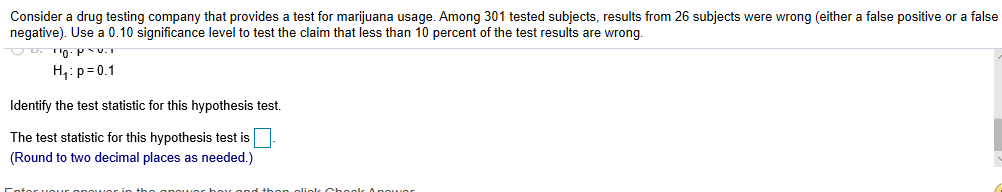Consider a drug testing company that provides a test for marijuana usage. Among 301 tested subjects, results from 26 subjects were wrong (either a false positive or a falsenegative). Use a 0.10 significance level to test the claim that less than 10 percent of the test results are wrong.H p 0.1Identify the test statistic for this hypothesis test.The test statistic for this hypothesis test is(Round to two decimal places as needed.)

Questionhelp_outlineImage TranscriptioncloseConsider a drug testing company that provides a test for marijuana usage. Among 301 tested subjects, results from 26 subjects were wrong (either a false positive or a false negative). Use a 0.10 significance level to test the claim that less than 10 percent of the test results are wrong. H p 0.1 Identify the test statistic for this hypothesis test. The test statistic for this hypothesis test is (Round to two decimal places as needed.) fullscreen
Step 1

Given hypothesis test
p be the proportion of wrong test results

Null hypothesis : p = 0.10
Alternate Hypothesis : p < 0.10

Sample size = n = 301
The number of wrong test results = 26
The proportion of wrong test results in sample = p^ = 26/301 = 0.08...

Want to see the full answer?

See Solution

Want to see this answer and more?

Our solutions are written by experts, many with advanced degrees, and available 24/7

See Solution
Tagged in

Hypothesis Testing